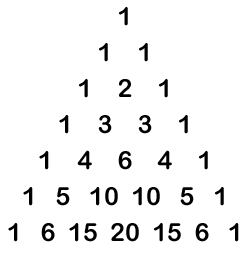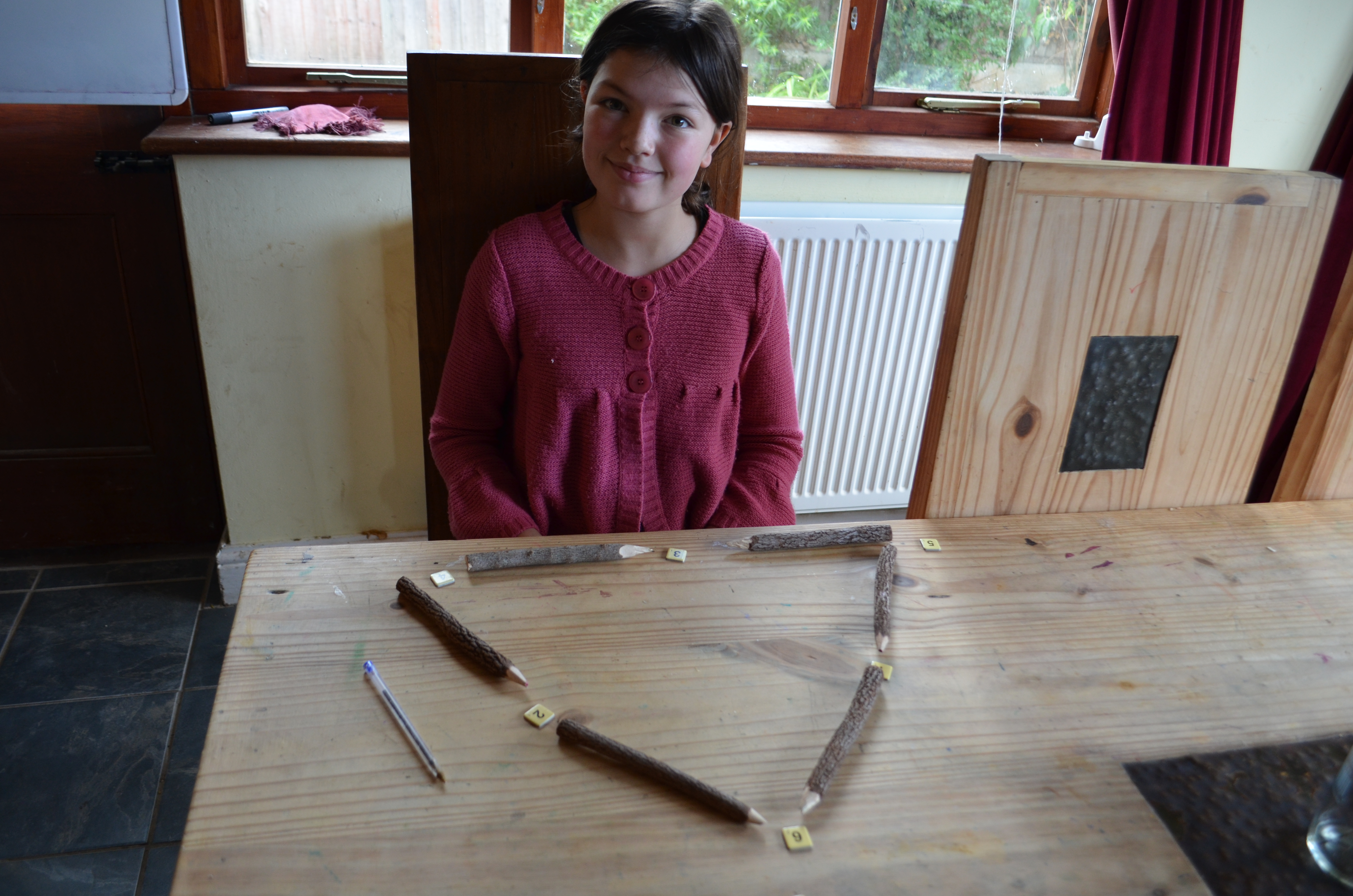# Helping a Struggling Maths Student: Pascal’s Triangle, Magic Triangles & Number Bond Games

Practising Number Bonds using Pascal’s Triangle

I thought I would have a fun frivolous lesson as both L10 and C10 seem to be handling any number bond related question I threw their way.  Pascal’s triangle is a triangle of numbers made up of lots of number bonds:The whole triangle fascinates me and I knew it would feature heavily in our future maths so I thought now would be an ideal time to introduce it.  This would not be an in-depth lesson looking at all the patterns.  That would come later.  No, this would simply be an introduction to the fun elements of maths. The one I gave them was much larger than the one above and went into much larger 3 digit numbers.  My goal was for the girls to look at a partially filled in one and see the number bond pattern for themselves.   The reality was they began to see all the other patterns first.  However, they picked up a pattern they assumed was correct but in fact wasn’t.  That was my chance to ask them to have a look for any number bonds.  It didn’t take long.  I then asked them to disprove the previous pattern they had been so sure of by filling in the rest of the numbers in Pascal’s triangle.  As the numbers got larger, I could see L10 beginning to use her fingers to count.  I hadn’t seen her do that all summer, so asked why?  She was struggling to do the larger numbers in her head.  I used this as an opportunity to give her some mental arithmetic strategies, some we had covered before others were new.

Mental Arithmetic Strategies

1. Count from largest to smallest amounts ie Add the hundreds first, then the tens then the units (this is completely counter to what she would normally do on paper)
2. Think in terms of placement, carrying if necessary.  So 367 +596 becomes 800+150+13 which is easier to calculate: 950+13 then 963
3. Adding large numbers is linked intrinsically to number bonds of ten and another method is to break down and put back together the numbers being added.  Using the same example above:

367+596=

300+500+60+40+50+7+3+3=

800+100+50+10+3=

900+60+3=

963

On paper these look cumbersome, but with instruction and practice one’s mind can do these almost imperceptibly in a matter of seconds.  I am a very visual learner, so in my head the numbers split up and re-join, dancing about, with very little effort required from me.  I am certain this would have taken time and effort to achieve when first learning mental arithmetic.  In teaching the girls these strategies and discouraging the use of finger counting, my goal was to begin the process of training their own brains to calculate without much thought.  Funnily enough C10 seems to do this naturally and doesn’t have too much of an issue with mental calculations.  For L10 it is, as everything mathematical seems to be, very difficult.  That said, she found calculating the smaller numbers much easier than she did five short weeks ago, so we are moving in the right direction.

Practising number bonds by using the Magic triangle

This is a fun activity whereby an equilateral triangle is cut and counters with 1-6 written are placed one in each corner and one in the middle of each triangle side.  The goal is to find a combination of numbers whereby the three numbers on each side of the triangle add up to the same as the other sides:Progressively larger triangles can be used, increasing the difficulty of the maths required to find the answer.

We started off simply, using equate tiles and wooden pencils for the numbers and triangle outlines.  I was pleased by how easy the girls found it:Number Bond Games

We had as family started to play games to reinforce all I was teaching.  Their success in our family came down to one thing.  If mummy or daddy were playing, it was seen as a great family games night.  If they weren’t involved, whilst there were no complaints, there was definitely less excitement.  As for Gary and I?  Our number bonds are coming along nicely, thank you very much!!

• Totally TutThis is a gentle game for 2-4 players and practises the four operations.  For now we just used it for addition and subtraction.

• EquateWe are huge fans of Scrabble in this household, so to find what is effectively a number Scrabble was quite exciting.  This is a maths facts game which can include fractions, decimals, whole numbers, addition, subtraction, multiplication and division.  It is easy to limit the facts practice to just whole numbers and addition and subtraction, which is what we are doing at the moment.  The rules are easy to follow and if you know how to play word Scrabble this is very similar.

• 21

This is a simple card game, which a friend taught us, and requires the individual to make up to a value of 21 with two or more cards.  Great for practicing addition facts.  You can find the rules here.  I am sure there are lots of other card games but we are not a family to play many card games so we are learning them as we go along or someone tells us about them.

• Sudoku

This is fairly new to our home-school, and while it doesn’t require adding or subtraction, it is number logic at its most simple, ensuring the children are playing with numbers and therefore increasing in confidence using them.  This website is particularly useful as it has a clear set of rules and tips as well as providing a daily printable Sudoku puzzle just for childrenI intend to print one each day for all of the older children to do when they have time on their hands, if they finish an activity early:We have already moved onto Place Value, which we are thoroughly enjoying.  I’ll be sharing that next week.